Question

In the rough approximation that the density of a planet is uniform throughout its interior, the gravitational field strength (force per unit mass) inside the planet at a distance from the center is , where is the radius of the planet. (For the Earth, at least, this is only a rough approximation, because the outer layers of rock have lower density than the inner core of molten iron). 1. Using the uniform-density approximation, calculate the amount of energy required to move a mass m from the center of the Earth to the surface. 2. For comparison, how much energy would be required to move the mass from the surface of the planet to a very large distance away? 3. Imagine that a small hole is drilled through the center of the Earth from one side to the other. Determine the speed of an object of mass m, dropped into this hole, when it reaches the center of the planet.

1.The answers to the questions are;

1. The amount of energy required to move a mass m from the center of the Earth to the surface is 0.5·m·g·R

2. The amount of energy required to move the mass from the surface of the planet to a very large distance away m·g·R.

3. The speed of an object of mass m, dropped into this hole, when it reaches the center of the planet is 9682.41783 m/s.

Explanation:

We note that the Work done W by the force F on the mass to move a small distance is given by

F×dr

The sum of such work to move the body to a required location is

W =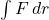F = mg’ = m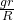We integrate from 0 to R (the center to the Earth surface)

Therefore W =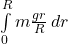Which gives W =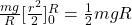2. To find the work done we have to integrate from the surface to infinity as follows W =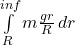=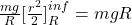The energy required to move the object to a large distance is equal to twice the energy reqired to move the object to the surface.

3 We note that the acceleration due to gravity at the surface is g and reduces to zero at the center of the Earth

v² = u² + 2·g·s

Radius of the Earth = 6371 km

From surface to half radius we have

v₁² = 2×9.81×6371/2×1000 = 62499460.04

v₁ = 7905.66 m/s

From the half the radius of the earth to the Earth center =

v₂² = 7905.66² + 2×9.81/2×6371/2×1000 = 93749215.04

v₂ = 9682.41783 m/s

The speed of an object of mass m, dropped into this hole, when it reaches the center of the planet. is 9682.41783 m/s